# 我国西南地区彝族人口空间分布研究Research on the Spatial Distribution of Yi People in Southwest China

DOI: 10.12677/OJNS.2018.64043, PDF, HTML, XML, 下载: 578  浏览: 3,332

Abstract: In this paper, the methods of median center, average center, central element, direction distribu-tion, standard distance, spatial autocorrelation and hot spot analysis are used to investigate and calculate the spatial distribution of Yi population in Southwest China. The results show that the central elements of Yi population in Southwest China are located in Yuxi City, the median center and average center are both located in Kunming City, and the Yi population in Southwest China has a positive spatial correlation, which is proportional to the degree of spatial aggregation. The spatial distribution of Yi population in this area is neither consistent with horizontal distribution nor vertical distribution, but the Yi population in southwest China has a high value spatial cluster in Yunnan province. That is to say, there is a high degree of confidence in the distribution of Yi population in this region, but in Chongqing and Guizhou Province, and very few areas in Sichuan Province, there is a low value spatial cluster in, that is, the distribution of Yi population in this region is relatively small.

1. 引言

2. 研究区域概况

2.1. 研究区域概况

2.2. 西南地区彝族概况

3. 数据来源、研究方法及技术路线

3.1. 数据来源及预处理

3.2. 研究方法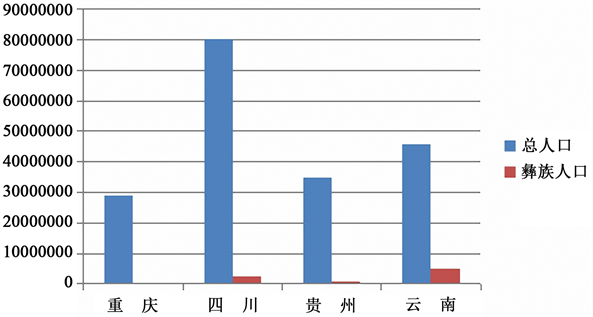Figure 1. The total population of Southwest China and the total population of Yi nationality

3.2.1. 中位数中心

${d}_{i}^{t}=\sqrt{{\left({X}_{i}-{X}^{t}\right)}^{2}+\left({Y}_{i}-{Y}^{t}\right)}$ (3-1)

3.2.2. 平均中心

$\stackrel{¯}{X}=\frac{\underset{i=1}{\overset{n}{\sum }}{X}_{i}}{n},\stackrel{¯}{Y}=\frac{\underset{i=1}{\overset{n}{\sum }}{Y}_{i}}{n}$ (3-2)

3.2.3. 中心要素

3.2.4. 方向分布

$SD{E}_{x}=\sqrt{\frac{\underset{i=1}{\overset{n}{\sum }}{\left({x}_{i}-\stackrel{¯}{X}\right)}^{2}}{n}}$ (3-3)

$SD{E}_{y}=\sqrt{\frac{\underset{i=1}{\overset{n}{\sum }}{\left({y}_{i}-\stackrel{¯}{Y}\right)}^{2}}{n}}$ (3-4)

$\mathrm{tan}\theta =\frac{A+B}{C}$ (3-5)

$A=\left(\underset{i=1}{\overset{n}{\sum }}{\stackrel{¯}{x}}_{i}^{2}-\underset{i=1}{\overset{n}{\sum }}{\stackrel{¯}{y}}_{i}^{2}\right)$ (3-6)

${\sigma }_{x}=\sqrt{2}\sqrt{\frac{\underset{i=1}{\overset{n}{\sum }}{\left({\stackrel{¯}{x}}_{i}\mathrm{cos}\theta -{\stackrel{¯}{y}}_{i}\mathrm{sin}\theta \right)}^{2}}{n}}$ (3-7)

$\sigma =\sqrt{2}\sqrt{\frac{\underset{i=1}{\overset{n}{\sum }}{\left({\stackrel{¯}{x}}_{i}\mathrm{sin}\theta +{\stackrel{¯}{y}}_{i}\mathrm{cos}\theta \right)}^{2}}{n}}$ (3-8)

3.2.5. 标准距离

$SD=\sqrt{\frac{\underset{i=1}{\overset{n}{\sum }}{\left({X}_{i}-{\stackrel{¯}{X}}_{i}\right)}^{2}}{n}+\frac{\underset{i=1}{\overset{n}{\sum }}{\left({Y}_{i}-{\stackrel{¯}{Y}}_{i}\right)}^{2}}{n}}$ (3-9)

3.2.6. 空间自相关分析

$I=\frac{n}{{S}_{o}}\frac{\underset{i=1}{\overset{n}{\sum }}\underset{j=1}{\overset{n}{\sum }}{w}_{i,j}{z}_{i}{z}_{j}}{\underset{i=1}{\overset{n}{\sum }}{z}_{i}^{2}}$ (3-10)

${S}_{o}=\underset{i=1}{\overset{n}{\sum }}\underset{j=1}{\sum }w{}_{i,j}$ (3-11)

${Z}_{I}=\frac{I-E\left[I\right]}{\sqrt{V\left[I\right]}}$ (3-12)

$E\left[I\right]=-1/\left(n-1\right)$ (3-13)

$V\left[I\right]=E\left[{I}^{2}\right]-E{\left[I\right]}^{2}$ (3-14)

3.2.7. 热点分析

${G}_{i}^{*}=\frac{\underset{j=1}{\overset{n}{\sum }}{w}_{i,j}{x}_{j}-\stackrel{¯}{x}\underset{j=1}{\overset{n}{\sum }}{w}_{i,j}}{S\sqrt{\frac{\left[n{\underset{j=1}{\overset{n}{\sum }}{w}_{i,j}^{2}-\left(\underset{j=1}{\overset{n}{\sum }}{w}_{i,j}\right)}^{2}\right]}{n-1}}}$ (3-15)

$\begin{array}{l}\stackrel{¯}{X}=\frac{\underset{j=1}{\overset{n}{\sum }}{x}_{j}}{n}\\ S=\sqrt{\frac{\underset{j=1}{\overset{n}{\sum }}{x}_{j}^{2}}{n}-{\left(\stackrel{¯}{X}\right)}^{2}}\end{array}$ (3-16)

3.3. 技术路线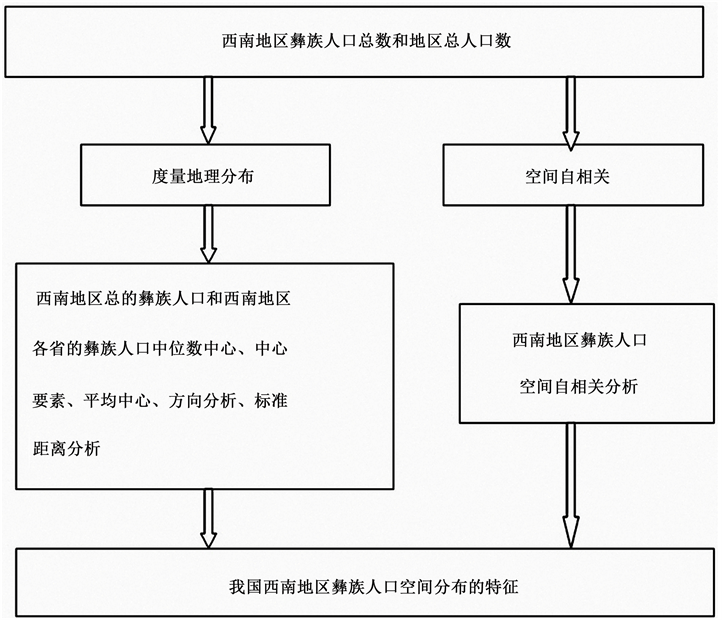4. 西南地区彝族人口空间分布状况分析

4.1. 西南地区彝族人口数状况

4.2. 西南地区总的彝族人口空间分布度量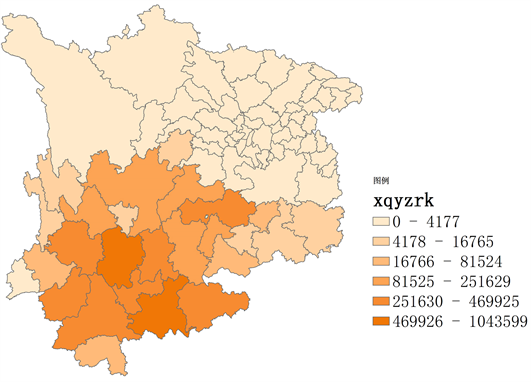Figure 3. The population of Yi nationality in Southwest China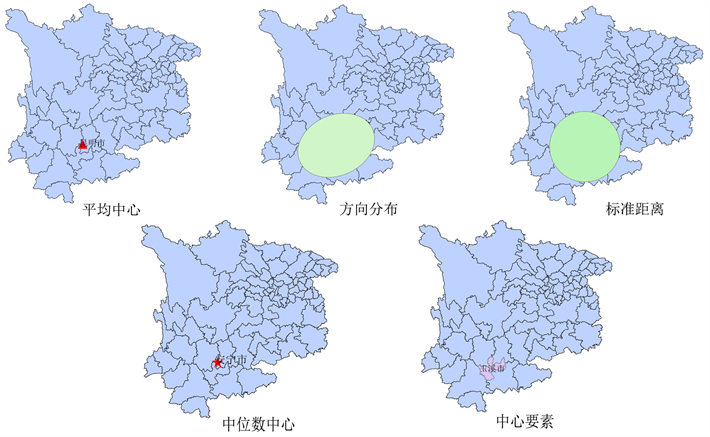Figure 4. Calculation results of Yi ethnic population in Southwest China

4.3. 西南地区各省的彝族人口空间分布度量

4.3.1. 云南省的彝族人口空间分布度量

4.3.2. 四川省的彝族人口空间分布度量

4.3.3. 贵州省的彝族人口空间分布度量

4.3.4. 重庆市的彝族人口空间分布度量

5. 西南地区彝族人口空间自相关及热点分析

5.1. 西南地区总的彝族人口空间自相关及热点分析

z得分为12.38，则随机产生此聚类模式的可能性小于1%。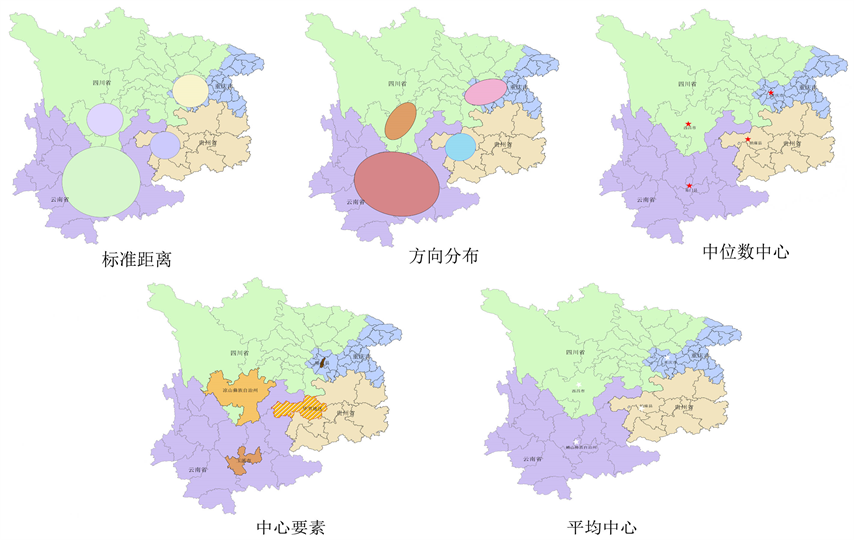Figure 5. Calculation results of Yi population in Southwest China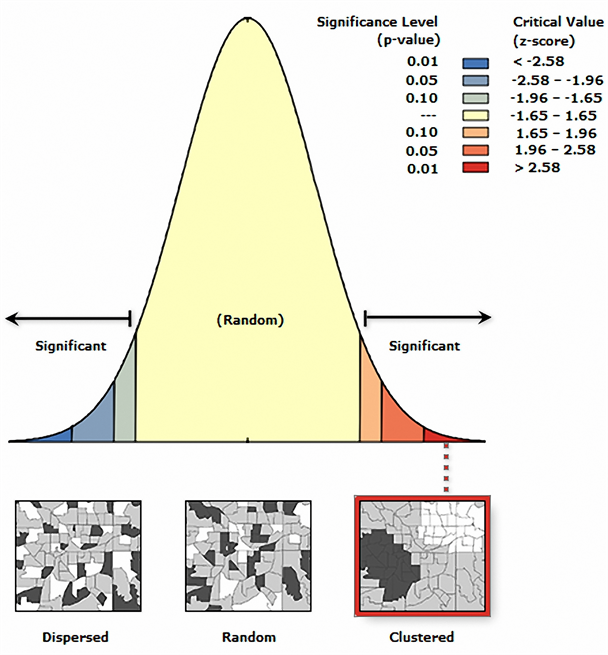Figure 6. Spatial autocorrelation analysis of Yi population in Southwest China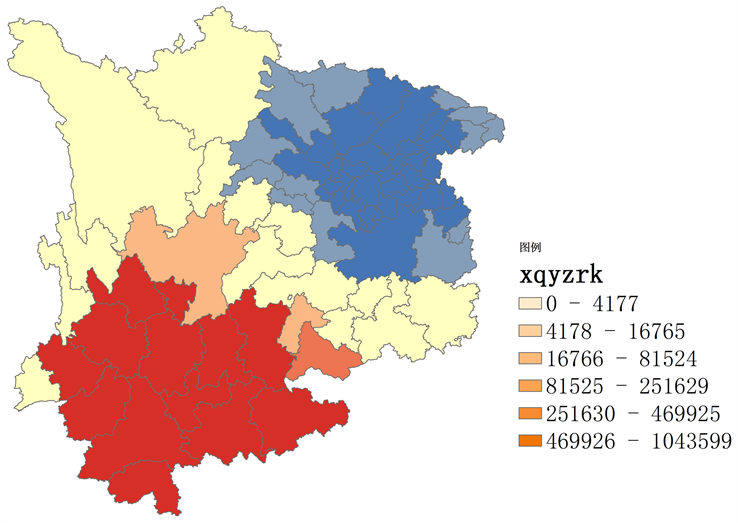Figure 7. Analysis of Yi population hotspots in Southwest ChinaTable 2. Spatial autocorrelation of Yi population in Southwest China

5.2. 西南地区各省的彝族人口空间自相关分析及热点分析

5.2.1. 云南省的彝族人口空间自相关分析及热点分析

Z得分为1.86，说明随机产生此聚类模式的可能性小于10%，又根据图表中的0.269178 > 0，说明该区域的彝族人口与空间聚集度成正比，空间相关性明显；Z得分为1.860285，p值为0.062845，95%的置信度，即该区域的聚类程度较大。又结合图8得出：云南省的彝族人口在玉溪市、文山壮族苗族自治州和红河哈尼族彝族自治州内存在一个高值的空间聚类，即该区域对彝族人口分布有着很高的置信度。玉溪市的置信度最高，即玉溪市及周边区域的彝族人口较多；文山壮族苗族自治州和红河哈尼族彝族自治州的置信度相对来说次之，即这两个州的周边有的区域分布着的彝族人口较少，所以它们两个州的置信度较低。

5.2.2. 四川省的彝族人口空间自相关分析及热点分析

Z得分为1.67，说明随机产生此聚类模式的可能性小于10%，又根据图表中的0.027789 > 0，说明该区域的彝族人口与空间聚集度成正比，空间相关性明显；Z得分为1.674757，p值为0.093982，90%的置信度，即四川省的彝族人口空间分布的聚类程度较大。结合图9得出：四川省的彝族人口在攀枝花市和凉山彝族自治州区域内存在一个高值的空间聚类，即该区域对彝族人口分布有着较高的置信度。且攀枝花市的置信度最高，即攀枝花市及周边区域分布着的彝族人口很多；凉山彝族自治州周边某些地区的彝族人口很少，即凉山彝族自治州的置信度相对较低。在德阳市、资阳市和遂宁市范围内存在一个低值的空间聚类，即该区域及周边地区分布着的彝族人口分布很少。

5.2.3. 贵州省的彝族人口空间自相关分析及热点分析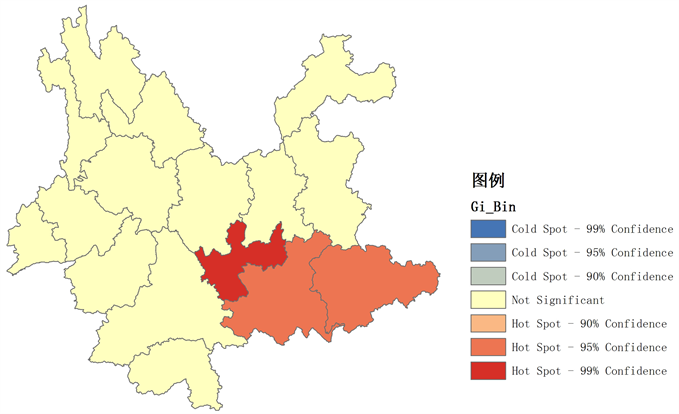Figure 8. Analysis of Yi population hotspots in Yunnan Province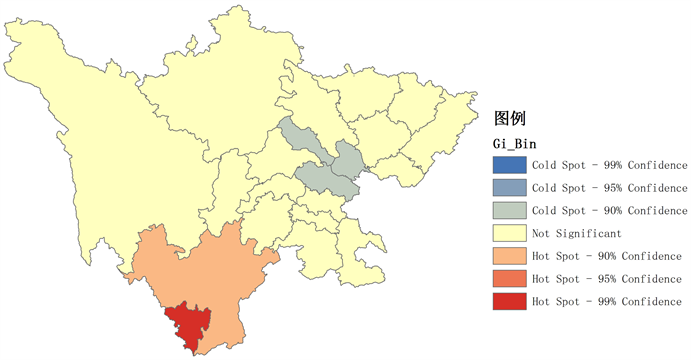Figure 9. Analysis of Yi population hotspots in Sichuan ProvinceTable 3. Spatial autocorrelation analysis of Yi population in Yunnan Province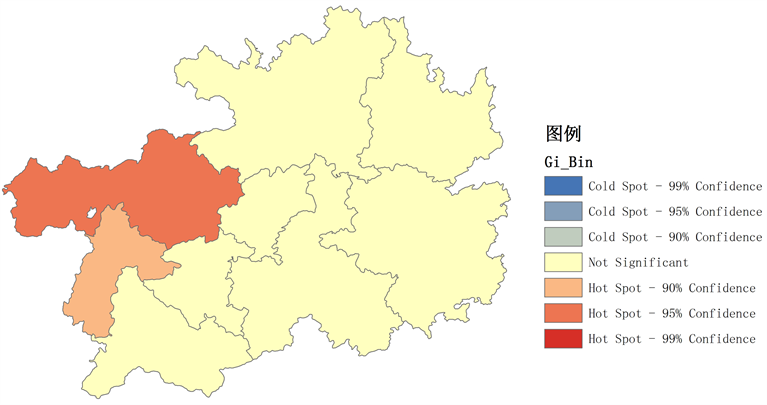Figure 10. Analysis of spatial autocorrelation of Yi population in Guizhou ProvinceTable 4. Spatial autocorrelation analysis of Yi population in Sichuan ProvinceTable 5. Spatial autocorrelation analysis of Yi population in Guizhou Province

Z得分为1.75，说明随机产生此聚类模式的可能性小于10%，又根据图表中的0.215977 > 0，说明该区域的彝族人口与空间聚集度成正比，空间相关性明显；Z得分为1.747911，p值为0.080479，90%的置度，即贵州省的彝族人口空间分布的聚类程度较大。结合图10得出：贵州省的彝族人口在毕节地区和六盘水市区域内存在一个高值的空间聚类，即该区域对彝族人口分布有着很高的置信度。且毕节地区的置信度最高，即毕节地区及周边区域分布着的彝族人口较多；六盘水市周边的有些区域彝族人口过少，故六盘水市的置信度相对较低。

5.2.4. 重庆市的彝族人口空间自相关分析及热点分析

Z得分为3.08，说明随机产生此聚类模式的可能性小于1%，又根据图表中的0.261954 > 0，说明该区域的彝族人口与空间聚集度成正比，空间相关性明显；Z得分为3.079061，p值为0.002077，99%的置信度，即重庆市的彝族人口空间分布的聚类程度较大。结合图11得出：重庆市的彝族人口在璧山县、合川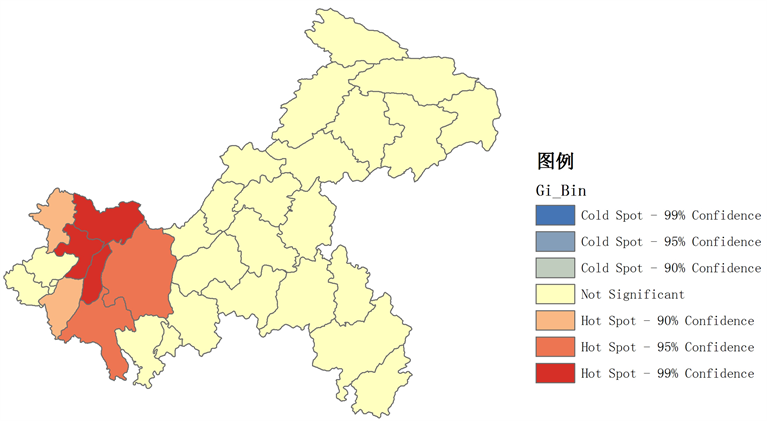Figure 11. Analysis of spatial autocorrelation of Yi population in ChongqingTable 6. Analysis of spatial autocorrelation of Yi population in Chongqing

6. 结论

1) 我国西南地区彝族人口中心要素位于玉溪市，中位数中心和平均中心皆位于昆明市；而西南地区其他四个省的彝族人口分布特征如下：云南省的中心要素位于玉溪市，中位数中心、平均中心皆位于昆明市；贵州省的三个参数的结果皆位于毕节地区；重庆市的中位数中心、平均中心皆位于重庆市，中心要素位于璧山县。

2) 我国西南地区的彝族人口具有空间正相关性，与空间聚集度成正比，且西南地区彝族人口空间分布的聚类程度较大，即我国西南地区的彝族人口较大部分是分布在云南省的；而西南地区其他四个省的彝族人口相关性如下：云南省的彝族人口与空间聚集度成正比，空间相关性明显且该区域的聚类程度较大；四川省的彝族人口与空间聚集度成正比，空间相关性明显且四川省的彝族人口空间分布的聚类程度较大；贵州省的彝族人口与空间聚集度成正比，空间相关性明显且贵州省的彝族人口空间分布的聚类程度较大；重庆市的彝族人口与空间聚集度成正比，空间相关性明显且重庆市的彝族人口空间分布的聚类程度较大。

3) 我国西南地区的彝族人口空间分布既不符合水平分布，也不符合竖直分布；云南省和贵州省的彝族人口空间分布同样不符合水平分布和竖直分布；四川省和重庆市的彝族人口空间分布都呈现水平分布状态。

4) 我国西南地区的彝族人口在云南省区域内有一个高值的空间聚类，即该区域对彝族人口分布有着很高的置信度，也就是云南省及周边区域分布着的彝族人口最多；在重庆市，以及贵州省、四川省的极少区域存在一个低值的空间聚类，即该区域的彝族人口分布较少；而西南地区其他四个省的彝族人口的空间聚类情况如下：云南省的彝族人口在玉溪市、文山壮族苗族自治州和红河哈尼族彝族自治州内存在一个高值的空间聚类，即该区域对彝族人口分布有着很高的置信度。玉溪市的置信度最高，即玉溪市及周边区域的彝族人口较多；文山壮族苗族自治州和红河哈尼族彝族自治州的置信度相对来说次之，即这两个州的周边有的区域分布着的彝族人口较少，所以它们两个州的置信度较低。四川省的彝族人口在攀枝花市和凉山彝族自治州区域内存在一个高值的空间聚类，即该区域对彝族人口分布有着较高的置信度。且攀枝花市的置信度最高，即攀枝花市及周边区域分布着的彝族人口很多；凉山彝族自治州周边某些地区的彝族人口很少，即凉山彝族自治州的置信度相对较低。在德阳市、资阳市和遂宁市范围内存在一个低值的空间聚类，即该区域及周边地区分布着的彝族人口分布很少。贵州省的彝族人口在毕节地区和六盘水市区域内存在一个高值的空间聚类，即该区域对彝族人口分布有着很高的置信度。且毕节地区的置信度最高，即毕节地区及周边区域分布着的彝族人口较多；六盘水市周边的有些区域彝族人口过少，故六盘水市的置信度相对较低。重庆市的彝族人口在璧山县、合川区、重庆市、铜梁县和江津区等区域内存在一个高值的空间聚类，即该区域对彝族人口分布有着很高的置信度。且合川区、璧山县和铜梁县的置信度最高，也就是说该区域及周边地区分布着的彝族人口较多；重庆市和江津区周边某些地区分布着的彝族人口较少，所以其置信度相对合川区等区域的置信度较低。

  张志斌, 杨莹, 张小平, 等. 我国西南地区风速变化及其影响因素[J]. 生态学报, 2014, 34(2): 471-481.  国政, 聂华, 臧润国, 等. 西南地区天然林保护工程生态效益评价[J]. 内蒙古农业大学学报(自然科学版), 2011, 32(2): 65-72.  董立峰, 王林林, 邵金花, 等. 近20年来山东省人口分布空间格局及其演变特征分析[J]. 国土与自然资源研究, 2012(3): 11-13.  王志伟. 中国历代人口分布空间化方法研究[D]: [硕士学位论文]. 兰州: 兰州大学, 2010.  江东, 杨小唤, 王乃斌, 等. 基于RS、GIS的人口空间分布研究[J]. 地球科学进展, 2002, 17(5): 734-738.  Clack, C. (1951) Urban Population Densities. Journal of the Royal Statistical Society, 114, 490-496. https://doi.org/10.2307/2981088  Clayton, C. and Estes, J. (1980) Image Analysis as Check on Census Enumeration Accuracy. Photogrammetric Engineering & Remote Sensing, 40, 757-764.  邓楚雄, 李民, 宾津佑. 湖南省人口分布格局时空变化特征及主要影响因素分析[J]. 经济地理, 2017(12): 41-48.  叶文振. 江西人口的地形区域分布: 1953-1993[J]. 南方人口, 1999(1): 47-52.  叶文振. 基于RS和GIS的江西省人口统计数据空间化: 1953-1993[J]. 江西科学, 2008, 26(6): 928-931.  叶明. 城市人口空间分析及其GIS应用模型[J]. 地域研究与开发, 2002, 21(2): 5-8.  胡焕庸. 论中国人口之分布[M]. 北京: 科学出版社, 1983.  高志强, 刘纪远, 庄大方. 基于遥感和GIS的中国土地资源生态环境质量同人口分布的关系研究[J]. 遥感学报, 1999, 3(1): 66-70.  王华东. 环境影响评价[M]. 北京: 高等教育出版社, 1989: 120-180.  云南省人民政府网. 云南省概况[EB/OL]. http://www.yn.gov.cn/yn_yngk/index.html, 2018-03-10.  重庆市人民政府网. 重庆概况[EB/OL]. http://www.cq.gov.cn/, 2018-03-10.  四川省人民政府网 .四川概况[EB/OL]. http://www.sc.gov.cn/, 2018-03-10.  贵州省人民政府网. 贵州概况[EB/OL]. http://www.gzgov.gov.cn/dcgz/gzgk/xzqh/index.html, 2018-03-10.  ArcGISPro [EB/OL]. http://pro.arcgis.com/zh-cn/pro-app/tool-reference/spatial-statistics/mean-center.htm, 2018-03-10.  Arc Resource Center [EB/OL]. http://help.arcgis.com/zh-cn/arcgisdesktop/10.0/help, 2018-03-10.